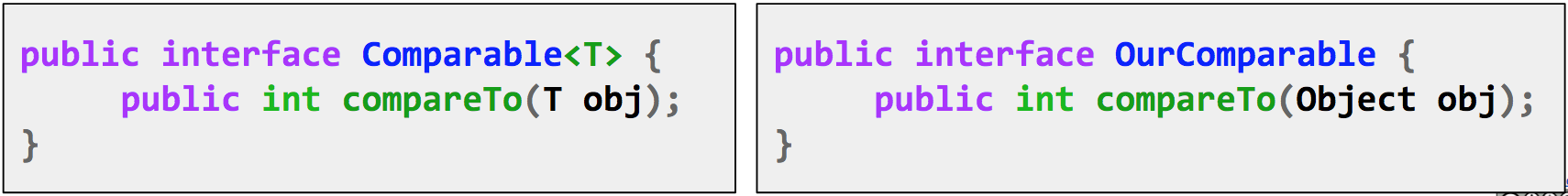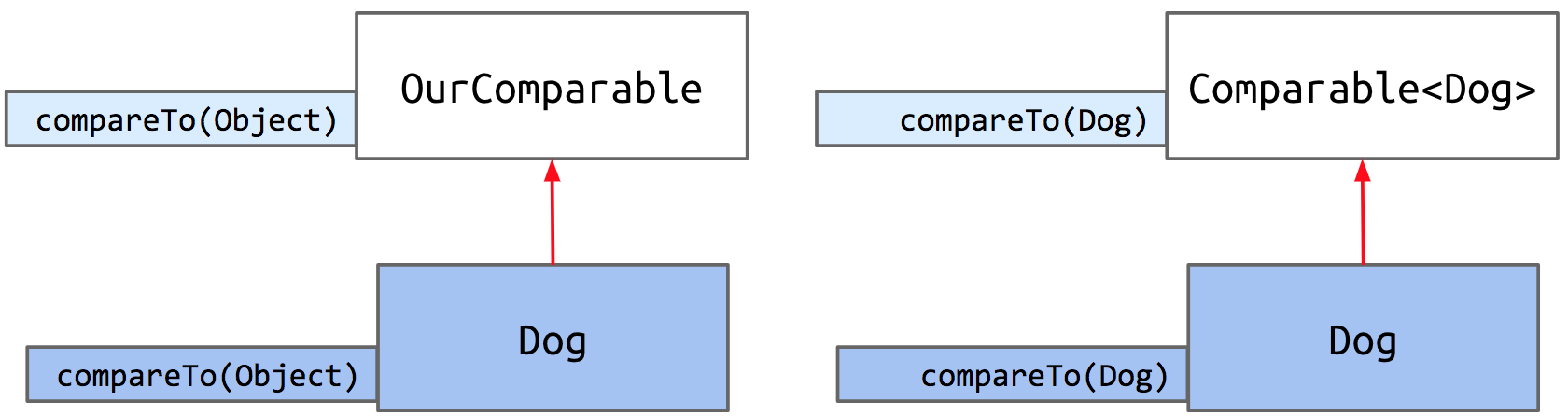## Subtype Polymorphism

We've seen how inheritance lets us reuse existing code in a superclass while implementing small modifications by overriding a superclass's methods or writing brand new methods in the subclass. Inheritance also makes it possible to design general data structures and methods using polymorphism.

Polymorphism, at its core, means 'many forms'. In Java, polymorphism refers to how objects can have many forms or types. In object-oriented programming, polymorphism relates to how an object can be regarded as an instance of its own class, an instance of its superclass, an instance of its superclass's superclass, and so on.

Consider a variable deque of static type Deque. A call to deque.addFirst() will be determined at the time of execution, depending on the run-time type, or dynamic type, of deque when addFirst is called. As we saw in the last chapter, Java picks which method to call using dynamic method selection.

Suppose we want to write a python program that prints a string representation of the larger of two objects. There are two approaches to this.

1. Explicit HoF Approach
def print_larger(x, y, compare, stringify):
if compare(x, y):
return stringify(x)
return stringify(y)

1. Subtype Polymorphism Approach
def print_larger(x, y):
if x.largerThan(y):
return x.str()
return y.str()


Using the explicit higher order function approach, you have a common way to print out the larger of two objects. In contrast, in the subtype polymorphism approach, the object itself makes the choices. The largerFunction that is called is dependent on what x and y actually are.

### Max Function

Say we want to write a max function which takes in any array - regardless of type - and returns the maximum item in the array.

Exercise 4.3.1. Your task is to determine how many compilation errors there are in the code below.

public static Object max(Object[] items) {
int maxDex = 0;
for (int i = 0; i < items.length; i += 1) {
if (items[i] > items[maxDex]) {
maxDex = i;
}
}
return items[maxDex];
}

public static void main(String[] args) {
Dog[] dogs = {new Dog("Elyse", 3), new Dog("Sture", 9), new Dog("Benjamin", 15)};
Dog maxDog = (Dog) max(dogs);
maxDog.bark();
}


In the code above, there was only 1 error, found at this line:

if (items[i] > items[maxDex]) {


The reason why this results in a compilation error is because this line assumes that the > operator works with arbitrary Object types, when in fact it does not.

Instead, one thing we could is define a maxDog function in the Dog class, and give up on writing a "one true max function" that could take in an array of any arbitrary type. We might define something like this:

public static Dog maxDog(Dog[] dogs) {
if (dogs == null || dogs.length == 0) {
return null;
}
Dog maxDog = dogs;
for (Dog d : dogs) {
if (d.size > maxDog.size) {
maxDog = d;
}
}
return maxDog;
}


While this would work for now, if we give up on our dream of making a generalized max function and let the Dog class define its own max function, then we'd have to do the same for any class we define later. We'd need to write a maxCat function, a maxPenguin function, a maxWhale function, etc., resulting in unnecessary repeated work and a lot of redundant code.

The fundamental issue that gives rise to this is that Objects cannot be compared with >. This makes sense, as how could Java know whether it should use the String representation of the object, or the size, or another metric, to make the comparison? In Python or C++, the way that the > operator works could be redefined to work in different ways when applied to different types. Unfortunately, Java does not have this capability. Instead, we turn to interface inheritance to help us out.

We can create an interface that guarantees that any implementing class, like Dog, contains a comparison method, which we'll call compareTo.Let's write our interface. We'll specify one method compareTo.

public interface OurComparable {
public int compareTo(Object o);
}


We will define its behavior like so:

• Return -1 if this < o.
• Return 0 if this equals o.
• Return 1 if this > o.

Now that we've created the OurComparable interface, we can require that our Dog class implements the compareTo method. First, we change Dog's class header to include implements OurComparable, and then we write the compareTo method according to its defined behavior above.

Exercise 4.3.2. Implement the compareTo method for the Dog class.

We use the instance variable size to make our comparison.

public class Dog implements OurComparable {
private String name;
private int size;

public Dog(String n, int s) {
name = n;
size = s;
}

public void bark() {
System.out.println(name + " says: bark");
}

public int compareTo(Object o) {
return -1;
} else if (this.size == uddaDog.size) {
return 0;
}
return 1;
}
}


Notice that since compareTo takes in any arbitrary Object o, we have to cast the input to a Dog to make our comparison using the size instance variable.

Now we can generalize the max function we defined in exercise 4.3.1 to, instead of taking in any arbitrary array of objects, takes in OurComparable objects - which we know for certain all have the compareTo method implemented.

public static OurComparable max(OurComparable[] items) {
int maxDex = 0;
for (int i = 0; i < items.length; i += 1) {
int cmp = items[i].compareTo(items[maxDex]);
if (cmp > 0) {
maxDex = i;
}
}
return items[maxDex];
}


Great! Now our max function can take in an array of any OurComparable type objects and return the maximum object in the array. Now, this code is admittedly quite long, so we can make it much more succinct by modifying our compareTo method's behavior:

• Return negative number if this < o.
• Return 0 if this equals o.
• Return positive number if this > o.

Now, we can just return the difference between the sizes. If my size is 2, and uddaDog's size is 5, compareTo would return -3, a negative number indicating that I am smaller.

public int compareTo(Object o) {
}


Using inheritance, we were able to generalize our maximization function. What are the benefits to this approach?

• No need for maximization code in every class(i.e. no Dog.maxDog(Dog[]) function required
• We have code that operates on multiple types (mostly) gracefully

### Interfaces Quiz

Exercise 4.3.3. Given the Dog class, DogLauncher class, OurComparable interface, and the Maximizer class, if we omit the compareTo() method from the Dog class, which file will fail to compile?

public class DogLauncher {
public static void main(String[] args) {
...
Dog[] dogs = new Dog[]{d1, d2, d3};
System.out.println(Maximizer.max(dogs));
}
}

public class Dog implements OurComparable {
...
public int compareTo(Object o) {
return -1;
} else if (this.size == uddaDog.size) {
return 0;
}
return 1;
}
...
}

public class Maximizer {
public static OurComparable max(OurComparable[] items) {
...
int cmp = items[i].compareTo(items[maxDex]);
...
}
}


In this case, the Dog class fails to compile. By declaring that it implements OurComparable, the Dog class makes a claim that it "is-an" OurComparable. As a result, the compiler checks that this claim is actually true, but sees that Dog doesn't implement compareTo.

What if we were to omit implements OurComparable from the Dog class header? This would cause a compile error in DogLauncher due to this line:

System.out.println(Maximizer.max(dogs));


If Dog does not implement the OurComparable interface, then trying to pass in an array of Dogs to Maximizer's max function wouldn't be approved by the compiler. max only accepts an array of OurComparable objects.

## Comparables

The OurComparable interface that we just built works, but it's not perfect. Here are some issues with it:

• Awkward casting to/from Objects
• No existing classes implement OurComparable (e.g. String, etc.)
• No existing classes use OurComparable (e.g. no built-in max function that uses OurComparable)

The solution? We'll take advantage of an interface that already exists called Comparable. Comparable is already defined by Java and is used by countless libraries.

Comparable looks very similar to the OurComparable interface we made, but with one main difference. Can you spot it?Notice that Comparable<T> means that it takes a generic type. This will help us avoid having to cast an object to a specific type! Now, we will rewrite the Dog class to implement the Comparable interface, being sure to update the generic type T to Dog:

public class Dog implements Comparable<Dog> {
...
}
}


Now all that's left is to change each instance of OurComparable in the Maximizer class to Comparable. Watch as the largest Dog says bark:

Instead of using our personally created interface OurComparable, we now use the real, built-in interface, Comparable. As a result, we can take advantage of all the libraries that already exist and use Comparable.## Comparator

We've just learned about the comparable interface, which imbeds into each Dog the ability to compare itself to another Dog. Now, we will introduce a new interface that looks very similar called Comparator.

Let's start off by defining some terminology.

• Natural order - used to refer to the ordering implied in the compareTo method of a particular class.

As an example, the natural ordering of Dogs, as we stated previously, is defined according to the value of size. What if we'd like to sort Dogs in a different way than their natural ordering, such as by alphabetical order of their name?

Java's way of doing this is by using Comparator's. Since a comparator is an object, the way we'll use Comparator is by writing a nested class inside Dog that implements the Comparator interface.

But first, what's inside this interface?

public interface Comparator<T> {
int compare(T o1, T o2);
}


This shows that the Comparator interface requires that any implementing class implements the compare method. The rule for compare is just like compareTo:

• Return negative number if o1 < o2.
• Return 0 if o1 equals o2.
• Return positive number if o1 > o2.

Let's give Dog a NameComparator. To do this, we can simply defer to String's already defined compareTo method.

import java.util.Comparator;

public class Dog implements Comparable<Dog> {
...
}

private static class NameComparator implements Comparator<Dog> {
public int compare(Dog a, Dog b) {
return a.name.compareTo(b.name);
}
}

public static Comparator<Dog> getNameComparator() {
return new NameComparator();
}
}


Note that we've declared NameComparator to be a static class. A minor difference, but we do so because we do not need to instantiate a Dog to get a NameComparator. Let's see how this Comparator works in action.

As you've seen, we can retrieve our NameComparator like so:

Comparator<Dog> nc = Dog.getNameComparator();


All in all, we have a Dog class that has a private NameComparator class and a method that returns a NameComparator we can use to compare dogs alphabetically by name.

Let's see how everything works in the inheritance hierarchy - we have a Comparator interface that's built-in to Java, which we can implement to define our own Comparators (NameComparator, SizeComparator, etc.) within Dog.To summarize, interfaces in Java provide us with the ability to make callbacks. Sometimes, a function needs the help of another function that might not have been written yet (e.g. max needs compareTo). A callback function is the helping function (in the scenario, compareTo). In some languages, this is accomplished using explicit function passing; in Java, we wrap the needed function in an interface.

A Comparable says, "I want to compare myself to another object". It is imbedded within the object itself, and it defines the natural ordering of a type. A Comparator, on the other hand, is more like a third party machine that compares two objects to each other. Since there's only room for one compareTo method, if we want multiple ways to compare, we must turn to Comparator.